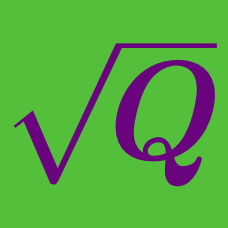Algebra

# Radical Expressions and Equations: Level 1 Challenges

$\Large 2^{\color{red}3} + 2^{\color{red}3} + 3^{\color{blue}4} + 3^{\color{blue}4} + 3^{\color{blue}4} = {\ \color{teal}} ?$

If half of $$\sqrt{2}$$ is $$\sqrt{k}$$, then what is the value of $$k?$$

$\large \sqrt{\frac{1}{\sqrt{ x}}}=3, \ \ \ x = \ ?$

$\large \frac{\sqrt{12}-\sqrt{27}}{6}\times\sqrt{3}\times\sqrt{4}= \ ?$

$\large\color{green} 6^{\color{blue} 6} + \color{green} 6^{\color{blue} 6} + \color{green} 6^{\color{blue} 6} + \color{green} 6^{\color{blue} 6} + \color{green} 6^{\color{blue} 6} + \color{green} 6^{\color{blue} 6} = \color{green}6^ {\color{brown}a}$

If $$\color{brown} a$$ satisfies the equation above, what is the value of $$\color{brown} a$$?

×

Problem Loading...

Note Loading...

Set Loading...